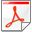## Zigzag Persistent Homology in Matrix Multiplication Time

Nikola Milosavljevic, Dmitriy Morozov, Primoz Skraba.
 Proceedings of the Annual Symposium on Computational Geometry, pages 216-225, 2011.SoCG'11
Abstract
We present a new algorithm for computing zigzag persistent homology, an algebraic structure which encodes changes to homology groups of a simplicial complex over a sequence of simplex additions and deletions. Provided that there is an algorithm that multiplies two n × n matrices in M(n) time, our algorithm runs in O(M(n) + n2 log2 n) time for a sequence of n additions and deletions. In particular, the running time is O(n2.376), by result of Coppersmith and Winograd. The fastest previously known algorithm for this problem takes O(n3) time in the worst case.
References

Gunnar Carlsson, Vin de Silva, and Dmitriy Morozov. Zigzag persistent homology and real-valued functions. Proceedings of the Annual Symposium on Computational Geometry, pages 247–256, 2009.